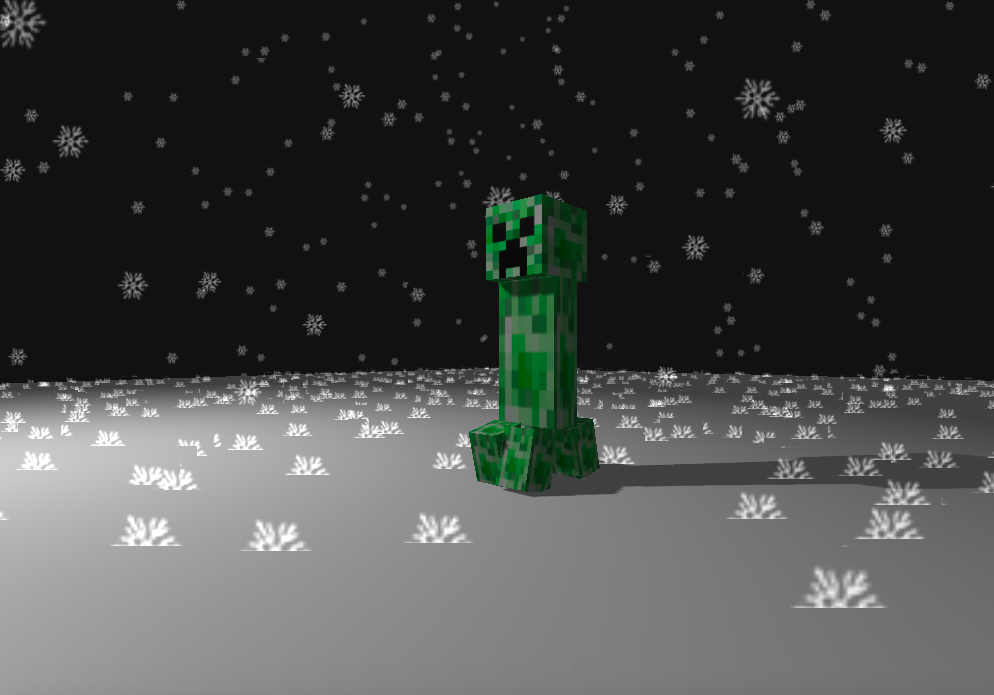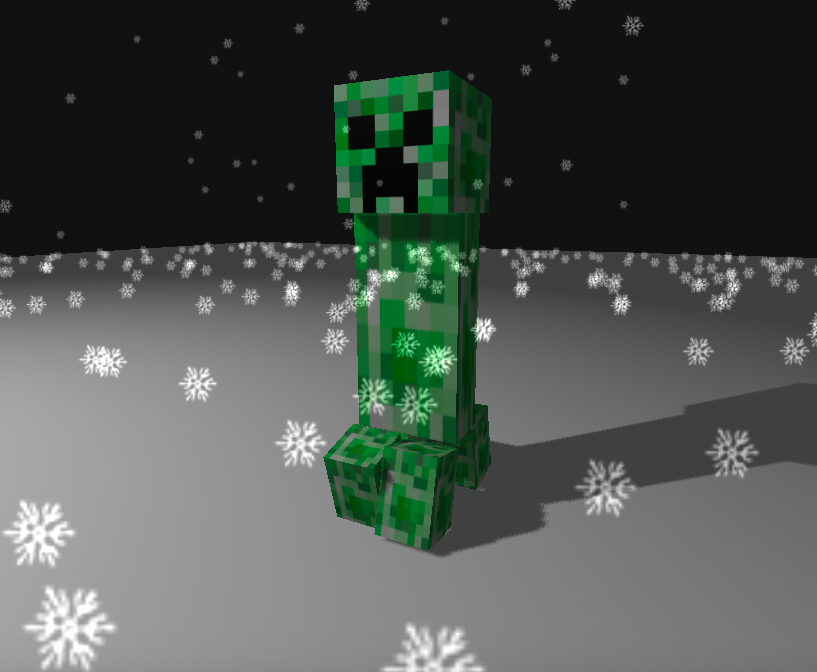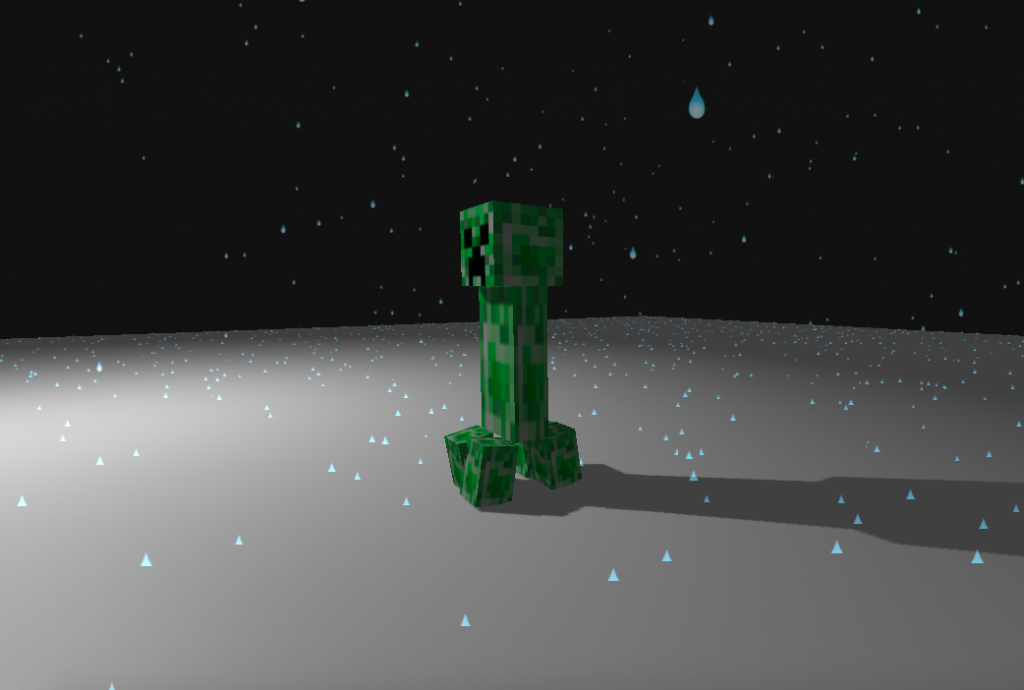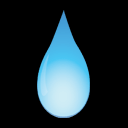#2019 iT 邦幫忙鐵人賽DAY 17
1
Modern Web

## 用 Three.js 來當個創世神 (16)：專案實作#8 - 粒子特效場景Photo by Fancycrave on Unsplash

## 今日目標## 專案實作

### 1. 整合動畫與雪花場景

``````// 粒子系統全域變數宣告
const particleCount = 15000
let points
let material
``````
``````// 粒子系統初始化
function createPoints() {
const geometry = new THREE.Geometry()

material = new THREE.PointsMaterial({
size: 5,
map: snowTexture,
// depthTest: false, // 遮蔽效果
transparent: true,
opacity: 0.5
})

const range = 300
for (let i = 0; i < particleCount; i++) {
const x = THREE.Math.randInt(-range / 2, range / 2)
const y = THREE.Math.randInt(0, range * 20)
const z = THREE.Math.randInt(-range / 2, range / 2)
const point = new THREE.Vector3(x, y, z)
point.velocityX = THREE.Math.randFloat(-0.16, 0.16)
point.velocityY = THREE.Math.randFloat(0.1, 0.3)
geometry.vertices.push(point)
}

points = new THREE.Points(geometry, material)
}
``````
``````// 粒子系統動畫
function pointsAnimation() {
points.geometry.vertices.forEach(function(v) {
if (v.y >= -7) {
v.x = v.x - v.velocityX
v.y = v.y - v.velocityY
}
if (v.x <= -150 || v.x >= 150) v.velocityX = v.velocityX * -1
})

points.geometry.verticesNeedUpdate = true
}
``````### 3. 背景音樂

``````const sound = document.querySelector('audio')
let musicPlayback = false
...
this.togglePlayMusic = function() {
if (musicPlayback) {
sound.pause()
musicPlayback = false
} else {
sound.play()
musicPlayback = true
}
}
``````

### 4. 雨水材質圖源

``````this.changeScene = function() {
if (sceneType === 'SNOW') {
material.map = rainTexture
material.size = 2
sceneType = 'RAIN'
} else {
material.map = snowTexture
material.size = 5
sceneType = 'SNOW'
}
}
``````